login  home  contents  what's new  discussion  bug reports help  links  subscribe  changes  refresh  edit

Errors in symbolic integration

Risch-Bronstein-Trager algorithm (Risch algorithm in short) is a complete algorithom for integration in terms of elementary functions. The algorithm either finds elementary integral or proves that there is none. Existence of elementary integral is relatively rare, so given random elementary function probably does not have elementary integral. FriCAS implementation of Risch algorithm is probably the "most complete" existing implementation. Unfortunatly "most complete" does not mean complete, some parts are still unimplemented. See RischImplementationStatus. Unlike some other systems FriCAS will not give you unevaluated result when hitting unimplemented part. Instead, it signals error with message indicating that given integral requires unimplemented part. So when FriCAS returns unevaluated result almost surely there is on elementary integral. Almost surely, because as all programs FriCAS may have bugs...

FriCAS in fact implements extension of Risch algorithm which extends class of integrands to some Liouvillian functions and for integration in terms of Ei, Ci, Si, li, erf, fresnelS, fresnelC, incomplete Gamma and polylog. There is complete extended algorithm for large class of functions (but polylog causes tricky theoretical problems). Compared to theory current FriCAS implementation contains considerable gaps. Nevertheless, FriCAS can handle a lot of examples involving special functions that no other system can handle.

Additionaly to Risch integrator FriCAS contains relativly weak pattern matching integrator which can generate a few special functions -- Ei, li, dilog and erf. However, if integral really requires elliptic functions then the best thing which FriCAS can do is to prove that integral is nonelementary.

FriCAS Examples

1)

fricas
(1) -> integrate(sin(x)+sqrt(1-x^3),x)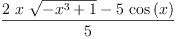(1)
Type: Union(Expression(Integer),...)

Here FriCAS proved that result is not elementary and found integral in terms of elliptic functions. Unfortunately, due to a bug during final processing this result got mangled and elliptic integral part is dropped, giving wrong result.

We gets correct result when we keep only algebraic part

fricas
integrate(sqrt(1-x^3),x)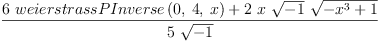(2)
Type: Union(Expression(Integer),...)

Reduce code

 int(sin(x)+sqrt(1-x^3),x); reduce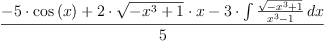2)

fricas
integrate(sqrt(1-log(sin(x)^2)),x)
>> Error detected within library code:
integrate: implementation incomplete (constant residues)

In this case FriCAS neither can compute elementry result nor can it prove that result is not elementary, is it gives up with error message indicating that the handling this integral requires unimplemented part of Bronstein-Trager algorithm.

 int(sqrt(1-log(sin(x)^2)),x); reduce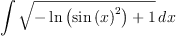3)

fricas
integrate(sqrt(sin(1/x)),x)
>> Error detected within library code:
integrate: implementation incomplete (has polynomial part)

Again, this integral needs unimplemented part of Bronstein-Trager algorithm.

 int(sqrt(sin(1/x)),x); reduce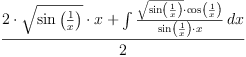4)

fricas
)set output tex off

fricas
)set output algebra on
integrate(sqrt(sin(x)),x)
(3)
+------+
+-+ | +---+
\|2 \|\|- 1
*
weierstrassZeta
4
,
0
,
+---+          +---+
- sin(x) + \|- 1 cos(x) + \|- 1
weierstrassPInverse(4,0,--------------------------------)
+---+          +---+
sin(x) + \|- 1 cos(x) + \|- 1
+
+--------+
+-+ |   +---+
\|2 \|- \|- 1
*
weierstrassZeta
4
,
0
,
+---+          +---+
- sin(x) - \|- 1 cos(x) - \|- 1
weierstrassPInverse(4,0,--------------------------------)
+---+          +---+
sin(x) - \|- 1 cos(x) - \|- 1
/
+--------+ +------+
|   +---+  | +---+
\|- \|- 1  \|\|- 1
Type: Union(Expression(Integer),...)

This time FriCAS can prove that result is nonelementary (it needs elliptic functions).

 int(sqrt(sin(x)),x); reduce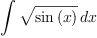For this Maple 9 gives the following result: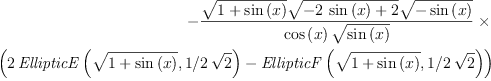(3)

And Mathematica 4 gives: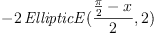(4)

symbolic integration
Tue, 22 Mar 2005 11:48:00 -0600 reply
fricas
)set output tex on

fricas
)set output algebra off
integrate(exp(-x^2),x)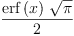(5)
Type: Union(Expression(Integer),...)
Errorfunction
Wed, 23 Mar 2005 08:23:21 -0600 reply
fricas
integrate(exp(-x^2/2)/sqrt(%pi*2),x=%minusInfinity..%plusInfinity)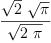(6)
Type: Union(f1: OrderedCompletion?(Expression(Integer)),...)

Works, roots remain unsimplified to preserve branches:

fricas
integrate(x^6*exp(-x^2/2)/sqrt(%pi*2),x=%minusInfinity..%plusInfinity)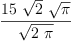(7)
Type: Union(f1: OrderedCompletion?(Expression(Integer)),...)
fricas
integrate(x^6*exp(-x^2/2)/sqrt(%pi*2),x)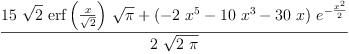(8)
Type: Union(Expression(Integer),...)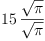(9)

... --unknown, Thu, 25 Aug 2005 05:57:53 -0500 reply
fricas
integrate(exp(x)/x^2,x)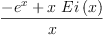(10)
Type: Union(Expression(Integer),...)

fricas
integrate(sqrt(x), x)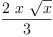(11)
Type: Union(Expression(Integer),...)

 Subject:   Be Bold !! ( 14 subscribers )